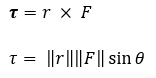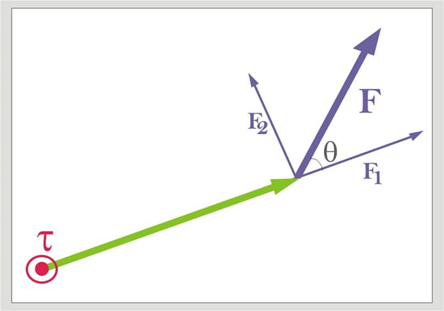# Angular Acceleration

## Angular Acceleration - Units, Equation and Examples

Angular acceleration is the rate at which angular speed changes or change in angular speed in unit time. Since it is in three dimensions, it is a pseudovector. In SI units, it is estimated in radians per second squared (rad/s2) and is generally depicted by the Greek letter alpha (α).

$\alpha = \frac{{d\omega }}{{dt}},$

where ω (omega) is the vector depicting angular velocity.

The angular acceleration of a given particle α can be connected to the torque(τ) being applied through the following equation:
$I\alpha = \tau ,$

where α is the angular acceleration of the particle and "I" is its moment of inertia.

Angular acceleration is a quantitative articulation of the change in angular speed that a turning object experiences for each unit time and it is also sometimes called as rotational acceleration. It is a vector amount, comprising of a range segment and both of the two characterized headings or faculties.

The extent, or length, of the angular acceleration vector, is, in particular, relative to the rate of progress of angular speed, and is estimated in radians per second squared (radians per second) 2 or (radians * second) - 2. On the other hand, the angular acceleration magnitude can be communicated in degrees per second squared (degrees per second) 2 or (degrees * second) - 2

The course of the angular acceleration vector is opposite to the plane in which the turn happens. If the increase in angular speed seems clockwise as per an eyewitness, at that point the angular acceleration vector points away from the onlooker. If the increase in angular acceleration seems counterclockwise, at that point the angular acceleration vector points towards the spectator.

The angular acceleration vector does not necessarily point along the same direction as the angular speed vector. Consider a vehicle moving forward along an expressway at increasing speed. The angular acceleration vectors for each of the four tires point toward the left along the lines containing the wheel axles. In the event that the vehicle quits acceleration and keeps up a steady speed, the angular acceleration vectors vanish. In the event that the vehicle backs off going ahead, the vectors turn around their headings and point toward the right, i.e., along with the lines containing the wheel axles. On the off chance that the vehicle is put into a reverse mode and increases speed while going in reverse, the angular acceleration vectors point toward the right, i.e., along with the lines containing the axles. On the off chance that the retrogressive speed is steady, the angular acceleration vectors disappear. If the regressive speed diminishes, the angular acceleration vectors point toward the left along the lines containing the wheel axles.

How is angular acceleration determined?

The angular acceleration of a turning object is the rate at which the angular speed changes with respect to the time taken. It is the adjustment in the angular speed, isolated by the adjustment in time. The normal angular acceleration is the adjustment in the angular speed, partitioned by the adjustment in time. The angular acceleration is a pseudovector that focuses toward a path along the turn pivot. The extent of the angular acceleration is given by the equation beneath. The unit of angular acceleration is radians/s2

$\alpha = \frac{{\Delta \omega }}{{\Delta t}} = \frac{{{\omega _2} - {\omega _1}}}{{{t_2} - {t_1}}}$

α = angular acceleration, (radians/s2)
Δω = change in angular speed (radians/s)
Δt = change in time (s)
ω1 = introductory angular acceleration (radians/s)
ω2= last angular speed (radians/s)
t1 = introductory time (s)
t2= last time (s)

What causes angular acceleration

In physics, when an all-encompassing article is pivoted, for example, a bar, plate, or 3D square, which has its mass appropriated through space, it is necessary to consider where the power is connected. This is where the concept of torque may be observed. Torque is a proportion of the volume of a power to cause a revolution. In physics terms, the torque applied on an item relies upon the power itself (its magnitude and direction) and where the power is applied. It goes from the entirely linear path of power as something that demonstrations in a straight line, (for example, when you drive a cooler up an incline) to its angular partner, torque.

Torque carries powers into the rotational world. Most items aren't simply tough and inflexible masses, so on the probability that they are pushed, they move as well as turn. For instance, in the event that you apply a power externally to a carousel, you don't move the carousel far from its present area — you cause it to begin turning.
The torque is a vector. The extent or magnitude of the torque discloses the capacity of the torque to create a revolution; all the more explicitly, the size of the torque is relative to the angular acceleration it produces. The bearing of the torque is along the hub of this angular acceleration.

Some important terms that are associated with angular acceleration:

• 1. Torque

• Torque, momentum, or moment of force is the variable which can be compared to linear force. The idea began with the investigations of Archimedes on the use of switches. Just as a linear force is a push or energy, a torque can be considered a twist to an article. The symbol for torque is normally ” τ”, the lowercase Greek letter tau. While being alluded to a moment of force, it is generally signified by M.

Since it is in three dimensions, the torque is a pseudovector. In the case of point particles, it is given by the cross result of the position vector (separate vector) and the power vector. The magnitude of torque of an inflexible body relies upon three variables: the force that is applied, the switch arm vector interfacing the root to the point of force application, and the angle between the power and switch arm vectors. In symbols:Where,
τ = torque produced
r = radius vector
F is the force vector perpendicular to the radius vector,

× refers to the cross product, which is characterized as sizes of the particular vectors times is the point between the power vector and the switch arm vector.

The SI unit for torque is N⋅m.

A force connected at a correct edge to a switch duplicated by its separation from the switch's support (the length of the switch arm) is its torque. A force of three newtons connected two meters from the support, for instance, applies indistinguishable torque from a force of one newton connected six meters from the support. The course of the torque can be controlled by utilizing the correct hand grasp rule: if the fingers of the right hand are twisted from the bearing of the switch arm to the heading of the power, at that point the thumb focuses toward the torque. The torque on a molecule (which has the position “r”) can be characterized as the cross product:

$\tau = r \times F,$

Where r is the position vector of the particle relative to the fulcrum, and F is the force that acts upon the particle.

The magnitude of torque is given by

$\tau = rF\sin \theta ,$

Where r is the distance between the axis of rotation and the particle, with F being the force applied and θ is the angle between the position vector and the force vector.• 1. Angular momentum

• In physics, angular momentum (sometimes, the moment of force or rotational force) is what might be compared to linear energy. It is a very important variable in physics since it is not always available in huge amounts —the absolute angular energy of a framework stays steady except if followed up on by an outer torque.

Since it is in three dimensions, the angular momentum for a point particle is a pseudovector(r × p), the cross result of the molecule's position vector r (with respect to some root) and its force vector p = mv. This definition can be connected to each point in the continuum like solids or liquids, or physical fields. In contrast to energy, angular force depends on where the root is situated since the molecule's position is estimated from it. The angular force vector of a point particle is parallel and usually corresponding to the angular speed vector ω (omega) of the molecule (how quick it’s angular position changes), where the steady of proportionality relies upon both the mass of the molecule and its separation from starting point. For consistent inflexible bodies, however, the turn angular acceleration ω (omega) is relative and may not be constantly parallel to the angular force of the article, making the balance of proportionality I (called the force of dormancy) a second-rank tensor instead of a scalar.

Angular energy is additive; the all-out angular force of a framework is the pseudovector aggregate of the angular momentum.

For continuums or fields, one uses reconciliation. The complete angular force of any inflexible body can be part into the whole of two primary segments: the angular energy of the focal point of mass (with a mass equivalent to the all-out mass) about the root, in addition to the turn angular energy of the article about the focal point of mass.

• 2. Angular velocity (speed)

• In physics, the angular velocity (speed) of a molecule is the rate at which it pivots around a picked focus point: that is, the time rate of progress of its angular dislodging with respect to the birthplace (in simple terms: how rapidly an item circumvents another item over some undefined time frame - for example how quick the earth circles the sun). It is estimated in edge per unit time, radians per second in SI units, and is typically depicted by the symbol omega (ω, rarely Ω). By tradition, positive angular velocity (speed) demonstrates counter-clockwise turn, while negative is clockwise.

Since it is three dimensions, angular acceleration is a pseudovector, with its scale (magnitude) estimating the rate of a pivot, and its heading pointing along the hub of revolution (opposite to the range and speed vectors). The up-or-down introduction of angular speed is ordinarily indicated by the right-hand rule.

Some real-life applications of angular acceleration:

• a) When quarterbacks throw the football, they insert a spin with their fingers, so that the ball spins swiftly as it flies through the air. Football fans define a good pass as a tight spiral.

• b) The pretty spiral pattern around the muzzle's exit hole are grooves which are cut into the barrel of a gun.

• c) Bullets which come out of a rifled barrel have grooves cut into them to have a spin on them when they are shot.